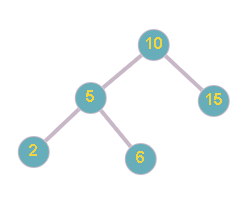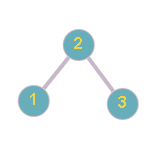6

Floor in BST

Difficulty: MEDIUM
Avg. time to solve
30 min
Success Rate
70%

Problem Statement

For example:In the above example, For the given BST  and X = 7, the greatest value node of the BST  which is smaller than or equal to  7 is 6.
Input Format:
The first line of input contains an integer ‘T’, denoting the number of test cases. Then each test case follows.

The first line of each test case contains nodes in level order form (separated by space). If any node does not have a left or right child, take -1 in its place.

The second and the last line of each test case contain integer ‘X’.

For example, the input for the tree depicted in the below image.10
5 15
2 6 -1 -1
-1 -1 -1 -1
Explanation :
Level 1 :
The root node of the tree is 10

Level 2 :
Left child of 10 = 5
Right child of 10 = 15

Level 3 :
Left child of 5 = 2
Right child of 5 = 6
Left child of 15 = null(-1)
Right child of 15 =  null (-1)

Level 4 :
Left child of 2 = null (-1)
Right child of 2 = null (-1)
Left child of 6 = null (-1)
Right child of 6 = null (-1)

The first not-null node(of the previous level) is treated as the parent of the first two nodes of the current level. The second not-null node (of the previous level) is treated as the parent node for the next two nodes of the current level and so on.
The input ends when all nodes at the last level are null(-1).
Note :
The above format was just to provide clarity on how the input is formed for a given tree.
The sequence will be put together in a single line separated by a single space. Hence, for the above-depicted tree, the input will be given as:
10 5 15 2 6 -1 -1 -1 -1 -1 -1
Output Format:
For each test case, print a single integer ‘M’, denoting the greatest value node of the BST  which is smaller than or equal to  ‘X’.

The Output of each test case will be printed on a separate line.
Note:
You do not need to print anything, it has already been taken care of. Just implement the given function.
Constraints:
1 <= T <= 5
1 <= N <= 5 * 10 ^ 3
1 <= nodeVal[i] <= 10 ^ 9

Time Limit: 1 sec.
Sample Input 1:
2
10 5 15 2 6 -1 -1 -1 -1 -1 -1
7
2 1 3 -1 -1 -1 -1
2
6
2
Explanation of Sample Input 1:
In the first test case, the BST looks like as below:The greatest value node of the BST which is smaller than or equal to  7 is 6.

In the second test case, the BST looks like as below:The greatest value node of the BST which is smaller than or equal to  2 is 2.
Sample Input 2:
2
5 3 10 2 4 6 15 -1 -1 -1 -1 -1 -1 -1 -1
15
5 3 10 2 4 6 15 -1 -1 -1 -1 -1 -1 -1 -1
8
Sample Output 2:
15
6Console